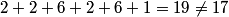## Solution to 1986 Problem 30

 (A) The n = 3 subshell consists of the l = 0 (s) subshell, the l = 1 (p) subshell, and the l = 2 (d) subshell. The l = 0 and the l = 1 subshell's are filled, but the l = 2 subshell is empty. So, (A) is false.(B) The 4s subshell corresponds to quantum numbers n = 4, l = 0. Two electrons with m = 0 can occupy this subshell, but the superscript indicates that there is only one electron, hence this subshell is not filled and (B) is false.(C) (D) The atomic number is the sum of the superscripts:$2+2+6+2+6+1 = 19 \neq 17$, thus (D) is false.(E)